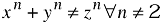# equation

equation — A displayed mathematical equation

## Synopsis

equation ::=

### Attributes

• floatstyle
• label
• pgwide (enumeration)
• “0”
• “1”

## Description

An `Equation` is a formal mathematical equation (with an optional rather than required title).

If the MathML Module is used, `Equation` can also contain the mml:math element.

### Processing expectations

Formatted as a displayed block. For an inline equation, use `InlineEquation`.

Processing systems that number equations or build a table of equations at the beginning of a document may have difficulty correctly formatting documents that contain both `Equation`s with `Title`s and `Equation`s without `Title`s. You are advised to use `InformalEquation` for equations without titles.

### Attributes

floatstyle

Specifies style information to be used when rendering the float

label

FIXME:

pgwide

Indicates if the element is rendered across the column or the page

Enumerated values:

0

The element should be rendered in the current text flow (with the flow column width).

1

The element should be rendered across the full text page.

### Children

The following elements occur in equation: `alt`, `caption` (db.caption), `info` (db.titleforbidden.info), `info` (db.titleonly.info), `mathphrase`, `mediaobject`, `mml:*`, `title`, `titleabbrev`.

## Examples

```<article xmlns='http://docbook.org/ns/docbook'>
<title>Example equation</title>

<equation xml:id="eq.fermat">
<title>Fermat's Last Theorem</title>
<!-- FIXME: alt broken in 5.0b1 <alt>x^n + y^n ≠ z^n ∀ n ≠ 2</alt> -->
<mediaobject>
<imageobject>
<imagedata fileref="figures/fermat.png"/>
</imageobject>
<textobject>
<phrase>x^n + y^n ≠ z^n ∀ n ≠ 2</phrase>
</textobject>
</mediaobject>
</equation>

</article>
```

Equation 1. Fermat's Last Theorem## ChangeLog

This alpha reference page is \$Revision: 1.5 \$ published \$Date: 2005/10/31 18:42:45 \$.